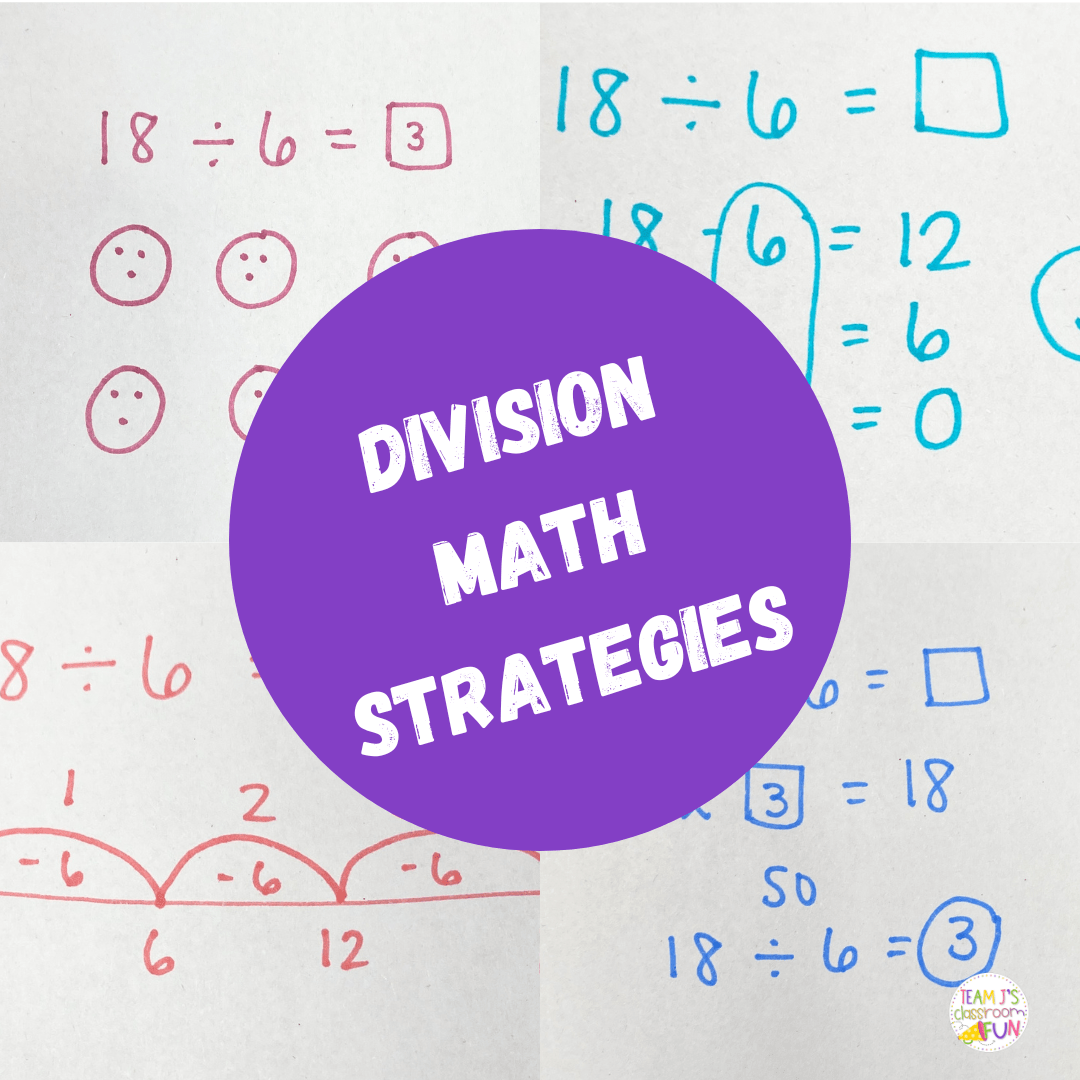Mathematics can sometimes feel like a tricky puzzle, especially when it comes to division strategies. Many students find division challenging and overwhelming, but fear not! There are fantastic division strategies that can make this math operation a whole lot easier and even enjoyable.

We’re going to explore some key division strategies that will help you build a strong foundation for math success. Whether you’re a parent or teacher searching for tools to support young learners, we’ve got you covered.

We’ll start with the basics and gradually move on to more advanced approaches. By mastering these division strategies, students can not only solve division problems accurately but also develop critical thinking skills, problem-solving abilities, and a deeper understanding of number relationships. These strategies lay the groundwork for more advanced mathematical concepts and serve as stepping stones toward mathematical fluency.

## Division Strategies

Below you will see five different division math strategies that your students might use.  Each student will be in a different spot with their math strategies. For example, some students need the concrete/manipulatives and equal groups will be best for them.  Other students might be ready for repeated subtraction or related facts.  Students can use a variety of strategies and will move through them at different rates when they are ready. I highly recommend letting them explore these strategies first so they understand the concept of division before having them memorize their division facts.

## Equal Groups

Draw out circles for the number of groups that you have. This problem is 18 divided into 6 groups and we need to figure out how many are in each group. Then, in each group put 1 dot until you get to 18. Finally, students will count how many are in each circle/group. Students can count these in a variety of ways. Some students may count by 1s, 2s, 4s. I recommend having them write the number they are on below each circle to help them keep track. After counting by 1’s, the students came to the answer of 3.

## Bar Diagram

This is very similar to equal groups just a different way to visually represent the problem. Students will draw out a rectangular bar and then divide that bar into 6 groups. Then, they will put one in each group until they get to the total number they started with – 18. Then, count how many are in each group. Some students might also like the visual with the number 3 instead of the dots depending on where they are in their understanding of division.

## Repeated Subtraction

Repeated subtraction is exactly like repeated addition except you’re subtracting. For this problem, students would start at 18 and would start subtracting by 6 until they get to 0. For example – 18-6 = 12, 12-6 = 6, and then 6-6 = 0. They had to subtract 6 – 3 different times so 3 is the quotient.

## Empty Number Line

The empty number line division strategy is similar to repeated subtraction just a different way of visualizing it. For students who like to use empty number lines in math, this strategy might be the best one for them. Students start by drawing their empty number line and placing the starting number 18 on the far right. Then, they subtract 6 until they get to 0. They had to subtract 6 – 3 times so the answer is 3.

## Related Facts

For math students who have a solid understanding of multiplication and are ready for a more abstract strategy – related facts might work for them. For this strategy, students turn the division problem 18/6 = ___ into a multiplication problem 6 x __ = 18. So now they are trying to figure out what they would multiply by 6 to get to 18 and then using that related fact to solve the division problem.

## Division Strategies Exit Ticket FREEBIE

Grab this FREE set of exit tickets that will provide your math students with a chance to practice and demonstrate their division strategies. Includes 3 half-page questions that make a great exit ticket, independent practice, or formative assessment. Click HERE for your Division Strategies FREEBIE.